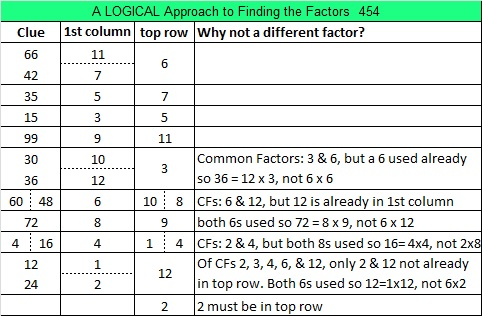# 454 and Level 5

There is a relationship between the digits in 454 and the digits of its two prime factors, specifically 4 + 5 + 4 = 13 and 2 + 2 + 2 + 7 = 13. That means 454 is another Smith number.

I am excited that Jo Morgan of Resourceaholic has awarded this blog the 2015 Resource Gem Award for Bright Ideas!Print the puzzles or type the factors on this excel file:  12 Factors 2015-04-06

——————————————————————————————————————-

• 454 is a composite number.
• Prime factorization: 454 = 2 x 227
• The exponents in the prime factorization are 1 and 1. Adding one to each and multiplying we get (1 + 1)(1 + 1) = 2 x 2 = 4. Therefore 454 has exactly 4 factors.
• Factors of 454: 1, 2, 227, 454
• Factor pairs: 454 = 1 x 454 or 2 x 227
• 454 has no square factors that allow its square root to be simplified. √454 ≈ 21.3073——————————————————————————————————————-This site uses Akismet to reduce spam. Learn how your comment data is processed.